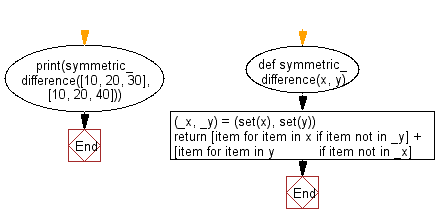﻿ Python: Return the symmetric difference between two iterables, without filtering out duplicate values - w3resource# Python: Return the symmetric difference between two iterables, without filtering out duplicate values

## Python List: Exercise - 242 with Solution

Write a Python program to get the symmetric difference between two iterables, without filtering out duplicate values.

• Create a set from each list.
• Use a list comprehension on each of them to only keep values not contained in the previously created set of the other.

Sample Solution:

Python Code:

``````def symmetric_difference(x, y):
(_x, _y) = (set(x), set(y))
return [item for item in x if item not in _y] + [item for item in y
if item not in _x]
print(symmetric_difference([10, 20, 30], [10, 20, 40]))
```
```

Sample Output:

```[30, 40]
```

Flowchart:## Visualize Python code execution:

The following tool visualize what the computer is doing step-by-step as it executes the said program:

Python Code Editor:

Have another way to solve this solution? Contribute your code (and comments) through Disqus.

What is the difficulty level of this exercise?

Test your Python skills with w3resource's quiz

﻿

## Python: Tips of the Day

Floor Division:

When we speak of division we normally mean (/) float division operator, this will give a precise result in float format with decimals.

For a rounded integer result there is (//) floor division operator in Python. Floor division will only give integer results that are round numbers.

```print(1000 // 300)
print(1000 / 300)```

Output:

```3
3.3333333333333335```